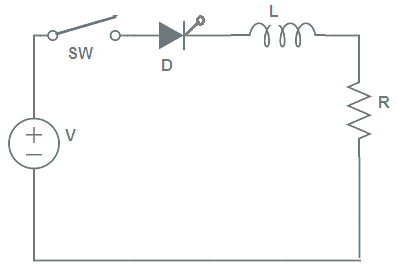# di/dt Protection of SCR Explained

di/dt protection is required to protect thyristor or SCR from the formation of local hot spot due to high value of rate of change of anode current i.e. di/dt near the gate to cathode junction during its turn on process.

When a thyristor or SCR is forward biased and is turned on by gate pulse, the conduction of anode current begins in the immediate neighborhood of the gate cathode junction. Thereafter, the anode current spread over the entire gate cathode junction area. The theyristor or SCR is designed in such a way to permit the speared of current in the entire junction are as soon as possible. But in case, the rate of rise of anode current di/dt is more than the spread velocity of the current in the junction area, then there will a chance for the formation of local hot spot. Since the current rises in the vicinity of the gate to cathode junction, hot spot may be formed in that region. This hot spot formation is harmful for the SCR and may damage it.This necessitates for a method of protection of SCR from high di/dt during turn on.

### Method of  di/dt Protection

The value of di/dt can be maintained below acceptable limit by using a small inductor in series with the anode circuit. This small inductor is called di/dt inductor.

### Calculation of Value of di/dt Inductor

Let us consider an SCR or thyristor having permissible value of di/dt of “S” A/µs. This SCR is controlling power through the Switch in a load resistance R. We are now required to find the value of di/dt inductor used to limit the di/dt within Q A/µs.When switch SW is closed and thyristor or SCR is turned on by gate pulse, the voltage equation for the circuit becomes

V = Ri + Ldi/dt

If we solve the above equation, the anode current will be given as below.

i = (V/R)[1  – e(-t/ τ)]

where τ = (L/R)

Differentiating the above value of current w.r.t time, we get

di/dt = (V/L)e(-t/ τ)

The maximum value of di/dt will occur just after the starting of conduction of SCR. Let this instant of time be t = 0. Therefore, the maximum value of di/dt will be given as

(di/dt)max = (V/L)

But maximum permissible value of di/dt of SCR is S, therefore

(di/dt)max ≤ S

(V/L) ≤ S

L ≥ (S/V)

Thus the minimum value of di/dt inductor should be equal to (S/V) where S is the permissible value of di/dt of SCR and V is the voltage source. If AC voltage source is used in place of DC, peak value of AC voltage i.e. Vm should be placed in the expression of L instead of V.

### 3 thoughts on “di/dt Protection of SCR Explained”

1.jorge barroso says:

On a VFD, how does this protection plays a role in the event of a short circuit downstream of the SCR, ie; short circuit in motor windings ? Is this protection faster than the fast acting fuses on the ac line side?

2.Danny Turner says:

Great explanation, but there is a typo in the last step. L should be greater than or equal to V/S, not S/V!

1.admin says:

Thank you for pointing out.

This site uses Akismet to reduce spam. Learn how your comment data is processed.